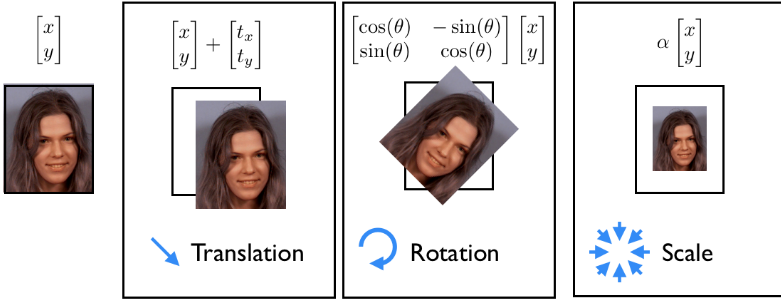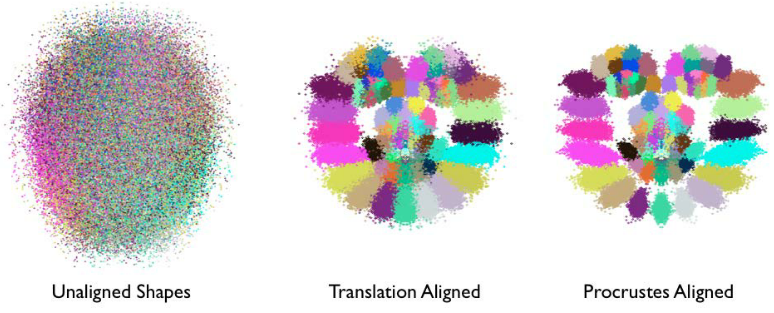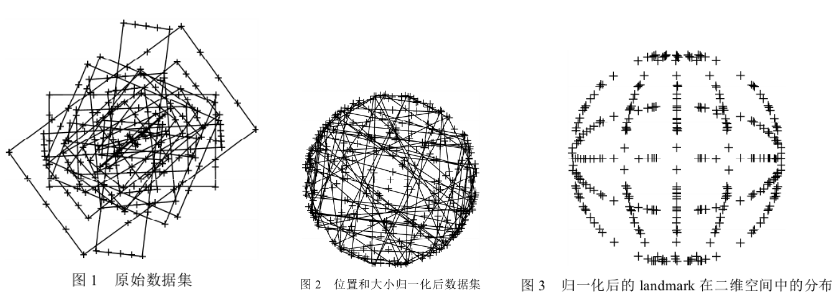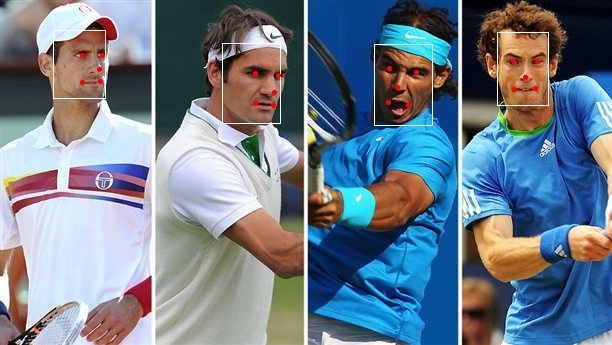# face alignment[Ordinary Procrustes Analysis]

• 人脸检测：从图片中准确定位到人脸，并以矩形框将其裁剪出来；
• 人脸矫正（对齐）： 检测到的人脸，可能角度不是很正，需要使其对齐，比如旋转，缩放；
• 特征提取：对矫正后的人脸进行特征提取，现在做法通常都是基于一个CNN模型；
• 人脸比对：对两张人脸图像提取的特征向量进行对比，计算相似度。

### 0 引言

MTCNN中采用的人脸矫正方法，是假设拿到的人脸几乎都是正脸，不过此时正脸有尺度不等，角度偏移等。而且需要预先设定一个平均脸，即目标脸的位置，标记出平均脸的所有关键点应该处于的位置，然后将所有人脸矫正到该平均脸上。

### 1 Procrustes Analysis普氏分析法

Facial geometry，通过两种元素的参数化配置组成：

• 全局变形（刚性）：指人脸在图像中的分布，允许人脸出现在图像中任意位置，包括人脸的坐标(x,y)、角度、大小；
• 局部变形（非刚性）：指不同人和不同表情之间脸部形状的不同，与全局形变不同，人脸的高度结构化特征对局部形变产生了极大的约束。• Procrustes analysis的作用可以看作是一种对原始数据的预处理，目的是为了获取更好的局部变化模型作为后续模型学习的基础。如下图所示:
• 每一个人脸特征点可以用一种单独的颜色表示；
• 经过归一化变化，人脸的结构越来越明显，即脸部特征簇的位置越来越接近他们的平均位置；
• 经过一系列迭代，尺度和旋转的归一化操作，这些特征簇变得更加紧凑，它们的分布越来越能表达人脸表情的变化。【剔除刚性部分、保留柔性部分】• 下图为不同大小、不同长宽比的矩形，经过归一化过程后，各个样本点分布服从一定概率分布趋势\begin{align} \begin{bmatrix} u \\ v \\ \end{bmatrix} & = \begin{bmatrix} a_2 & a_1 & a_0 \\ b_2 & b_1 & b_0 \\ \end{bmatrix} \begin{bmatrix} x \\ y \\ 1 \\ \end{bmatrix} \\ & = s\begin{bmatrix} cos\theta & -sin\theta \\ sin\theta & cos\theta \\ \end{bmatrix} \begin{bmatrix} x \\ y \\ \end{bmatrix}+\begin{bmatrix} t_1 \\ t_2 \\ \end{bmatrix} \\ & = sR\begin{bmatrix} x \\ y \\ \end{bmatrix}+T \end{align}

$Loss = \sum_i^N||sRp_i^T+T-q_i^T||^2$

\begin{aligned} & \underset{s,R,T}{\arg min}||sRp^T+T-q^T||_F \\ & s.t. \, R^TR=I \end{aligned}

\begin{aligned} & \underset{R}{\arg min}||RA-B||_F \\ & s.t. \, R^TR=I \end{aligned}

\begin{aligned} & M = BA^T \\ & svd(M) = U\Sigma V^T \\ & R = UV^T \\ \end{aligned}

### 2 代码

switching-eds-with-python第一部分所述：

• 将矩阵数值类型转换成float；
• 每个特征点减去当前形状的中心点，消除平移的影响（一旦找到了处理后的特征点集和的最优缩放因子和角度，这里的中心点c1和c2可以用来找回完整解）；
• 每个特征点除以当前形状的尺度因子，消除尺度的缩放影响；
• 使用SVD计算旋转角度；
• 返回一个仿射变换矩阵的完整矩阵。
'''a.py
https://matthewearl.github.io/2015/07/28/switching-eds-with-python/ 中代码是有问题的；

def transformation_from_points(points1, points2):
'''0 - 先确定是float数据类型 '''
points1 = points1.astype(numpy.float64)
points2 = points2.astype(numpy.float64)

'''1 - 消除平移的影响 '''
c1 = numpy.mean(points1, axis=0)
c2 = numpy.mean(points2, axis=0)
points1 -= c1
points2 -= c2

'''2 - 消除缩放的影响 '''
s1 = numpy.std(points1)
s2 = numpy.std(points2)
points1 /= s1
points2 /= s2

'''3 - 计算矩阵M=BA^T；对矩阵M进行SVD分解；计算得到R '''
# ||RA-B||; M=BA^T
A = points1.T # 2xN
B = points2.T # 2xN
M = np.dot(B, A.T)
U, S, Vt = numpy.linalg.svd(M)
R = np.dot(U, Vt)

'''4 - 构建仿射变换矩阵 '''
s = s2/s1
sR = s*R
c1 = c1.reshape(2,1)
c2 = c2.reshape(2,1)
T = c2 - np.dot(sR,c1) # 模板人脸的中心位置减去 需要对齐的中心位置（经过旋转和缩放之后）

trans_mat = numpy.hstack([sR,T])   # 2x3

return trans_mat


'''上述函数实现的时候，注意模板脸和需要对其的人脸的顺序
landmarks1： 检测出来需要对齐的人脸关键点；
landmarks2：对齐的模板人脸，即平均脸关键点'''

trans_mat = transformation_from_points(landmarks1,  landmarks2)

def warp_im(in_image, trans_mat, dst_size):
output_image = cv2.warpAffine(in_image,
trans_mat,
dst_size, # (cols, rows)
borderMode=cv2.BORDER_TRANSPARENT)
return output_image


### 3 例子'''边界框[x1, y1, x2, y2, score] '''
[222.22601686,  43.14613463, 280.12375677, 123.65308259, 1.        ],
[ 53.22718975,   30.1167623 ,  105.30491075,  98.62653339,   0.99999237],
[374.89622349,  44.23550975, 432.30359537, 125.07026242,  0.99998629],
[497.07639685,  32.2071521 ,  548.87478065, 105.17269786,  0.99970442]

'''points 关键点[x1, x2 ... x5, y1, y2 ..y5] '''
[255.76176 , 278.4415  , 274.27048 , 255.08255 , 273.5981  , 73.90924 ,  75.331924,  92.13313 , 103.375435, 105.174866],
[ 82.20165 , 102.67773 ,  99.4637  ,  82.03625 , 100.24012 ,  55.405067,  55.737637,  69.84144 ,  82.090454,  81.33897 ],
[389.51086 , 416.09406 , 397.90732 , 388.24335 , 408.37756 ,  68.65562 ,  72.8951  ,  87.913956, 102.8963  , 105.9071  ],
[513.3108  , 537.25714 , 525.7555  , 514.9328  , 535.16156 , 61.96994 ,  61.56072 ,  77.64981 ,  89.027435,  88.96181 ]



def extract_image_chips1( img, points, desired_size=256, padding=0):
"""
crop and align face
Parameters:
----------
img: numpy array, input image
points: numpy array, n x 10 (x1, x2 ... x5, y1, y2 ..y5)
desired_size: default 256
Retures:
-------
crop_imgs: list, n
cropped and aligned faces
"""
crop_imgs = []
for ind,p in enumerate(points):
# 当前图片中一共有len(points)个人脸
shape  =[]
for k in range(len(p)//2):
shape.append(p[k])
shape.append(p[k+5])

else:
# 平均脸（模板脸）的5个关键点坐标
mean_face_shape_x = [0.224152, 0.75610125, 0.490127, 0.254149, 0.726104]
mean_face_shape_y = [0.2119465, 0.2119465, 0.628106, 0.780233, 0.780233]

from_points = []
to_points = []

for i in range(len(shape)//2):
x = (padding + mean_face_shape_x[i]) / (2 * padding + 1) * desired_size
y = (padding + mean_face_shape_y[i]) / (2 * padding + 1) * desired_size
to_points.append([x, y])
from_points.append([shape[2*i], shape[2*i+1]])

# 构建人脸关键点矩阵
from_mat = np.asarray(from_points)
to_mat = np.asarray(to_points)

# 计算from_mat映射到to_mat的仿射变换矩阵，是一个2x3的矩阵
trans_mat = transformation_from_points(from_mat,to_mat)

# 进行仿射变换，并取当前中心向外(desired_size,desired_size)大小的区域
dst_size = (desired_size,desired_size)
chips = warp_im(img, trans_mat, dst_size)

crop_imgs.append(chips)

return crop_imgs

chips = extract_image_chips1(img, points, 144, 0.37)

对应的四个旋转矩阵为
array([[ 1.64844171e+00,  8.79546584e-02, -3.74864686e+02],
[-8.79546584e-02,  1.64844171e+00, -4.19148490e+01]])
array([[ 1.84494424e+00, -1.19245336e-02, -9.78834105e+01],
[ 1.19245336e-02,  1.84494424e+00, -4.60283424e+01]])
array([[ 1.48460564e+00,  2.23927075e-01, -5.41851323e+02],
[-2.23927075e-01,  1.48460564e+00,  4.27248780e+01]])
array([[ 1.79634282e+00, -3.48299747e-03, -8.71374899e+02],
[ 3.48299747e-03,  1.79634282e+00, -5.51812653e+01]])conference：

posted @ 2018-12-03 20:10  仙守  阅读(3873)  评论(0编辑  收藏  举报